# Rate of Change: Precalculus Lesson Plans Chapter Exam

Exam Instructions:

Choose your answers to the questions and click 'Next' to see the next set of questions. You can skip questions if you would like and come back to them later with the yellow "Go To First Skipped Question" button. When you have completed the practice exam, a green submit button will appear. Click it to see your results. Good luck!

Answered 0 of 30

### Page 1

#### Question 3 3. At how many points on the graph below is the derivative of f(x)=0?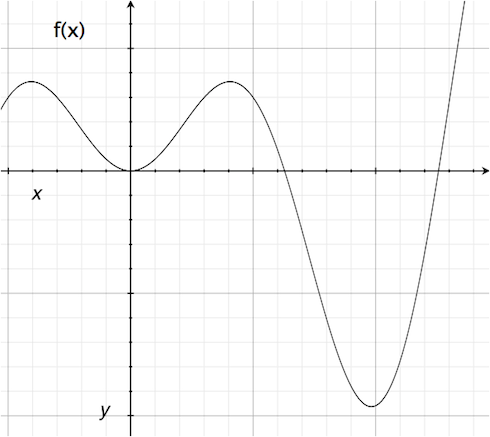#### Question 5 5. Where is f(x) differentiable?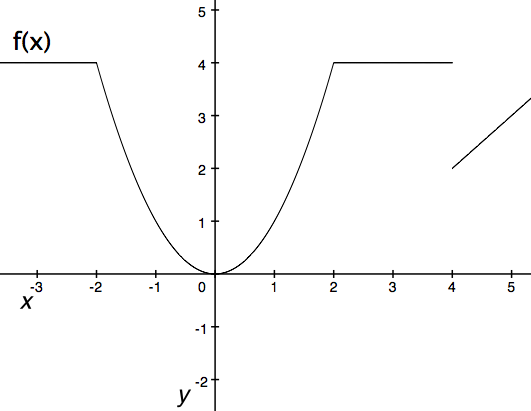### Page 2

#### Question 6 6. What is the rate of change at the point E in the graph below (for y in relation to x)?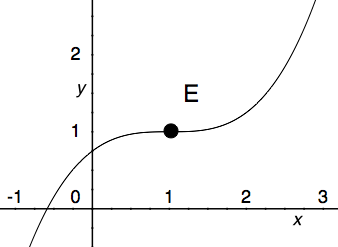#### Question 7 7. According to the mean value theorem, at how many points will the instantaneous rate of change equal the average rate of change between x = 1 and x = 3 on the following graph?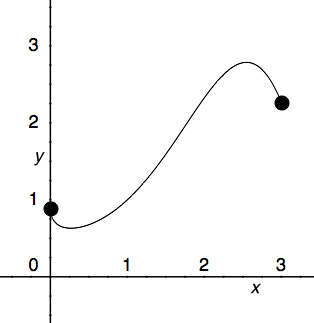#### Question 9 9. What is the rate of change at the point B in the graph below (for y in relation to x)?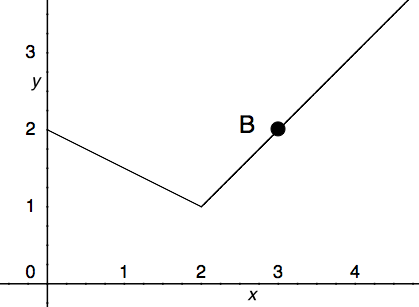#### Question 10 10. The derivative of f(x) is 0 at how many points on the graph below?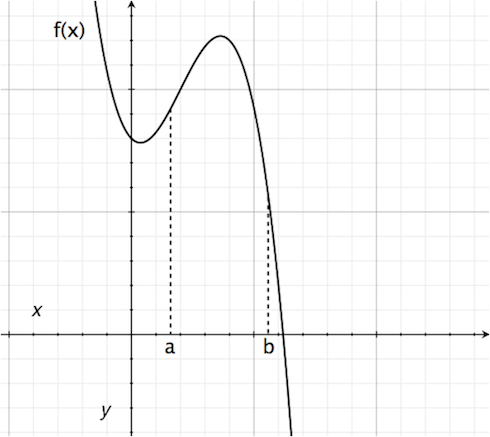### Page 3

#### Question 12 12. What is the average rate of change for the interval with endpoints A and B?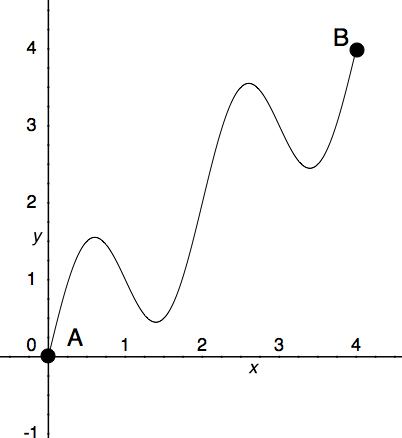#### Question 13 13. Consider the function f(x) given below. According to Rolle's theorem, for how many values of x is the instantaneous rate of change equal to 0?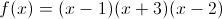### Page 4

#### Question 17 17. What is the value of f(x) at the red circle?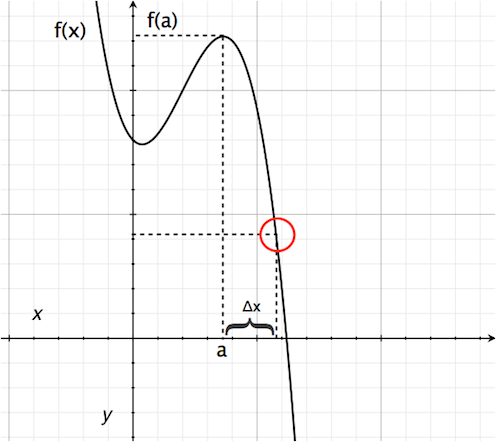#### Question 18 18. Consider the graph and question below..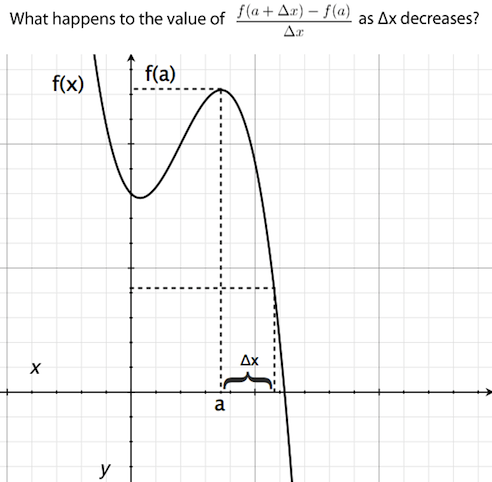#### Question 19 19. What is the rate of change at the point A in the graph below (for y in relation to x)?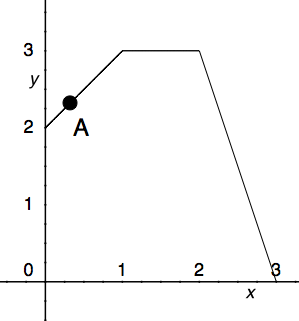#### Question 20 20. What is the derivative of the following?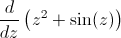### Page 5

#### Question 21 21. What is the rate of change at the point B in the graph below (for y in relation to x)? (In other words, what is the slope of the line from x = 2 to x = 3?)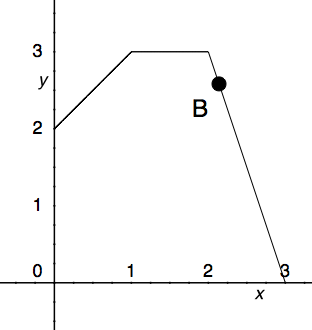#### Question 22 22. Where is f(x) differentiable?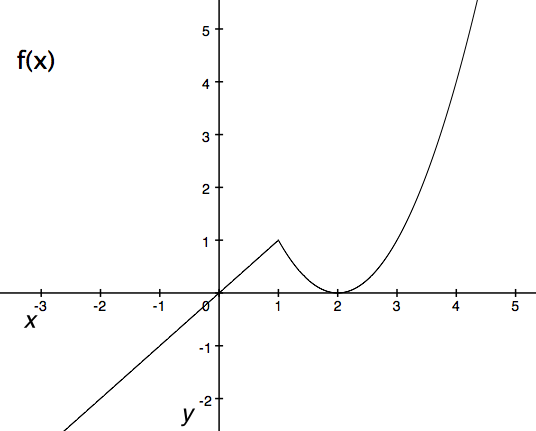#### Question 24 24. What is the approximate instantaneous rate of change at point C?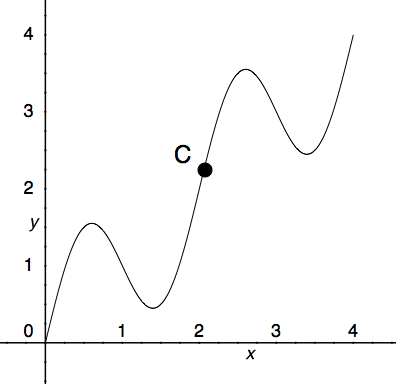#### Question 25 25. Consider the function f(x) given below. According to Rolle's theorem, between x=0 and x=pi, for how many values of x is the instantaneous rate of change equal to 0?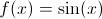### Page 6

#### Question 26 26. Consider the graph below. Between x=C and x=-C, for any value of C, will there be a point where the instantaneous rate of change is 0?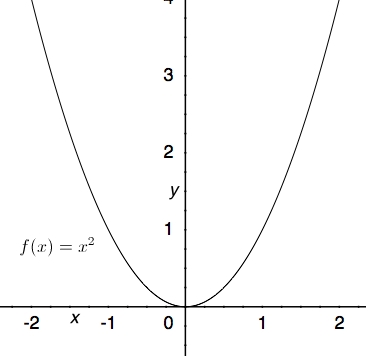#### Question 27 27. Where is y differentiable?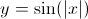#### Question 29 29. Where is f(x) differentiable?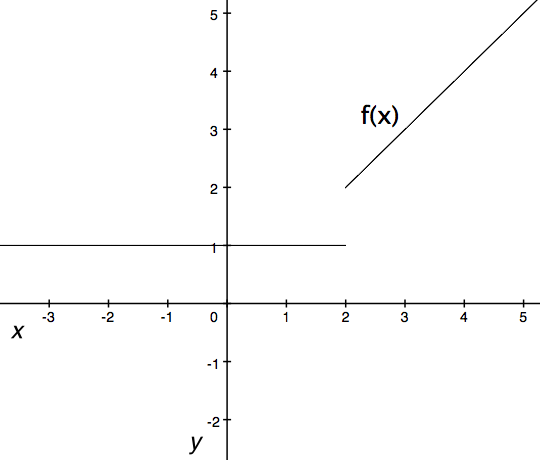#### Question 30 30. Which is greater, the derivative at a or the derivative at b?#### Rate of Change: Precalculus Lesson Plans Chapter Exam Instructions

Choose your answers to the questions and click 'Next' to see the next set of questions. You can skip questions if you would like and come back to them later with the yellow "Go To First Skipped Question" button. When you have completed the practice exam, a green submit button will appear. Click it to see your results. Good luck!

Support